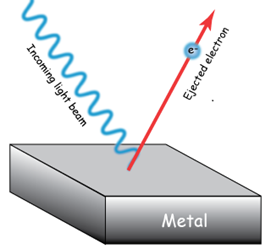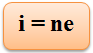×#### Thank you for registering.

One of our academic counsellors will contact you within 1 working day.

Click to Chat

1800-1023-196

+91-120-4616500

CART 0

• 0

MY CART (5)

Use Coupon: CART20 and get 20% off on all online Study Material

ITEM
DETAILS
MRP
DISCOUNT
FINAL PRICE
Total Price: Rs.

There are no items in this cart.
Continue Shopping• Complete Physics Course - Class 11
• OFFERED PRICE: Rs. 2,968
• View Details

Laws of Photoelectric Emission

Table of Content

Laws of Photo-Electricity

Determination of Photoelectric Current

Problem (JEE Main)

Related Resources“Photoelectric effect is the phenomenon of emission of electrons from the surfaces of certain substances, mainly metals, when light of shorter wavelength is incident upon them”.

Laws of Photo-Electricity

(a) Photoelectric effect is an instantaneous process.

(b) Photoelectric current is directly proportional to the intensity of incident light and is independent of frequency.

(c) The stopping potential and hence the maximum velocity of the electrons depends upon the frequency of incident light and is independent of its intensity.

(d) The emission of electrons stops below a certain minimum frequency known as threshold frequency.If P (in Watts) be the power of the source of radiation and I be the intensity of light radiation at a distance R from the source (R is the perpendicular distance of the receiving surface from the source), then:

I = P/4πR2

If radiation is falling on a plate of area A, then, energy absorbed/sec by the plate is given as:

Energy / sec = I × A = (P / 4πR2 × A ) J/sec

If v be the frequency of the source (i.e. light radiation), then energy per photon = h

So, number of photons failing on plate per second = energy per second / energy per photon.

Or, n/sec = P / 4πR2 × A / h             (Energy / photon = h = hc/λ)

If  > 0 (0: threshold frequency of plate) and photon efficiency of plate be x %, then the number of photoelectron emitted per second is:

n/sec (P/4πR2 × A / h) ×  x/100

Watch this Video for more reference

Determination of Photoelectric CurrentLet P be the power of a point source of electromagnetic radiations, then intensity I at distance r from the source is given by

I = P/4πr2 (W/m2)

If A is the area of a metal surface on which radiations are incident, then the power received by the plate is

P' = IA = P/4πr2 (W)

If f is the frequency of radiation, then the energy of photon is given by

E = hf

The number of photons incident on the plate per second (called photon flux) is given by

Φ  = P' / E = [P/4πr2 × A / hf]

If f > f0 (threshold frequency) and photon efficiency of the metal plate is η%, then the number of photoelectrons emitted per second is given by

n = Φη/100 [P/4πr2 × A / hf]] η/100Finally, the photocurrent i is given by,

Where e is the charge of an electron (e = 1.6 × 10–19 J)

Problem (JEE Main)

Radiation of two photons having energies twice and five times the work function of a metal are incident successively on the metal surface. Find out the ratio of the maximum velocity of photoelectrons emitted in the two cases.

Solution:

In the first case,

1/2 mv12 = 2W-W = W

So, v1 =√ (2W/m)

In the second case,

1/2 mv22 = 5W-W = 4W

So, v2 =√ (8W/m)

Thus, the ratio of the maximum velocity of photoelectrons emitted in the two cases will be, v1/v2 = ½ .

____________________________________________________________________________________________

Problem:

Photoelectric threshold of metallic silver is λ = 3800 Å. Ultra-violet light of λ = 2600 Å is incident on silver surface. Calculate

(a) the value of work function in joule and in eV.

(b) maximum kinetic energy of the emitted photoelectrons.

(c) the maximum velocity of the photo electrons (mass of the electron = 9.11 x 10–31 kg)

Solution:

(a) λ0 = 3800 Å

W = hf0 = h c/λ0 = 6.633 × 10–34 × 3 × 108 / 3800 × 10–10

J = 6.63 × 10–34 × 3 × 108 / 3800 × 10–10    J = 5.23 × 10–19        J = 3.27 eV

(b) Incident wavelength λ = 2600 Å

f = incident frequency = 3 × 108 / 2600 × 10–10 Hz

Then Tmax = hf – W0

hf = 6.63 × 1034 × 3 × 108 / 2600 × 10–10 = 6.63 × 3 / 2.6 × 10–19 J = 7.65 × 10–19 J = 4.78 eV

Tmax = hf – W0 = 4.78 eV – 3.27 eV = 1.51 eV.

(c) Tmax = 1/2 mv2max

Thus, vmax = √2Tmax / m = √2 × 2.242 × 10–19 / 9.11 × 10–31 = 0.7289 × 106 ms–1

_________________________________________________________________________________________________________________

Problem:

Sun gives light at the rate of 1400 Wm–2 of area perpendicular to the direction of light. Assume λ(sun light) = 6000 Å. Calculate the

(a) number of photons/sec arriving at 1m2 area at that part of the earth, and

(b) number of photons emitted from the sun/sec assuming the average radius of Earth's orbit is 1.49 x 1011 m.

Solution:

I = 1400 W / m2;       I = 6000 A

(a) n/sec = A / E/photon = (1400 × 1) × (6000 × 10–10) / 6.63 × 10–34 × 3 × 108 = 4.22 × 1021

(b) n/sec = Power of Sun(W) / E/photon = I × (4R2) × (6000 × 10–10) / 6.63 × 10–34 × 3 × 108 = 1.178 × 1045

___________________________________________________________________________________________________

Problem (JEE Main):

An isolated hydrogen atom emits a photon of 10.2 eV. Calculate:

(a) momentum of photon emitted

(b) recoil momentum of the atom

(c) kinetic energy of the recoil atom. Mass of proton = Mp = 1.67 x 10–27 kg.

Solution:

(a) momentum (p) = E/c

So, p = 10.2 × 1.6 × 10–19 / 3 × 108 = 5.44 × 10–27 kg m/s

(b) recoil momentum of atom = p (Law of conservation of momentum)

Thus, recoil momentum = 5.44 x 10–27 kg m/s

(c) KE = 1/2 mv2 (v = recoil speed of atom, m = mass of H-atom)

Thus, KE = 1/2 (p/m)2 = p2/2m

Therefore, KE = (5.44 × 10–27)2 / (2 × 1.67 × 10–27) = 8.86 × 10–27 JFor a light of any given frequency, photoelectric current is directly proportional to the intensity of light, provided the frequency is above the threshold frequency.

For a given material, there is a certain minimum (energy) frequency, called threshold frequency, below which the emission of photoelectrons stops completely, no matter how high is the intensity of incident light.

The maximum kinetic energy of the photoelectrons is found to increase with increase in the frequency of incident light, provided the frequency exceeds the threshold limit. The maximum kinetic energy is independent of the intensity of light.

The photo-emission is an instantaneous process. After the radiation strikes the metal surface, it just takes 10-9 s for the ejection of photoelectrons.

Photo-electric current falls to zero for some negative potential of opposite electrode. This negative potential is called stopping potential.

Photoelectric current stops below a particular frequency of incident light. The frequency is known as threshold frequency.

Photoelectric-effect establishes the quantum nature of radiation. This can be considered to be a proof in favour of particle nature of light.Question 1

When a metallic surface is illuminated with light of wave length , the stopping potential is V. When the same surface is illuminated by light of wavelength 2, the stopping potential is V/3. The threshold wavelength for the surface is,

(a) 4/3               (b) 4

(c) 6                  (d) 8/3

Question 2

Photons with energy 5 eV are incident on a cathode C, on a photoelectric cell. The maximum energy of the emitted photoelectrons is 2 eV. When photons of energy 6 eV are incident on C, no photoelectrons will reach the anode A if the stopping potential of A relative to C is,

(a) 3 V                   (b) -3 V

(c) -1 V                  (d) 4 V

Question 3

The photoelectric work function of a surface is 2.2 eV. The maximum kinetic energy of photo electrons emitted when light of wave length 6200 A.U. is incident on the surface is
(a) 1.6 eV              (b) 1.4 eV

(c) 1.2 eV              (d) photo electrons are not emitted

Question 4

A photo cell is illuminated by a point source of light 50cm away. When the source is shifted to 2m, then

(a) each emitted electron carries a quarter of the initial kinetic energy

(b) The number of electrons emitted is a quarter of the initial number

(c) each emitted electron carries one sixteenths the initial kinetic energy

(d) the number of electrons emitted is one sixteenths the initial number (e) the number of electrons emitted and the energy of each electron are one sixteenths the initial values.

Question 5

The retarding potential required to stop the emission of photoelectrons when a photosensitive material of work function 1.2 eV is irradiated with ultraviolet rays of wave length 2000 A.U. is
(a) 4V                   (b) 5V

(c) 6V                   (d) 8VQ.1
Q.2
Q.3
Q.4
Q.5

b

b

d

d

b

Related Resources

You might like to refer Emission of Electrons.

For getting an idea of the type of questions asked, refer the  Previous Year Question Papers.### Course Features

• 101 Video Lectures
• Revision Notes
• Previous Year Papers
• Mind Map
• Study Planner
• NCERT Solutions
• Discussion Forum
• Test paper with Video Solution